The Federation is the oldest and only Jewish Women's
Federation in the Country - Since 1916

*ALL MEMBERS OF OUR CONSTITUENT ORGANIZATIONS

ARE MEMBERS OF FEDERATION

<<<<<<<<<<<<<<<<<<<<<<<<<<<<<<<<<<<<<<<<<<<<<<<<<<<<<<<<<<<Visit and Like us on Facebook to Stay Informed!

<<<<<<<<<<<<<<<<<<<<<<<<<<<<<<<<<<<<<<<<<<<<<<<<<<<<<<<<<<<
Next Meeting
Mid-Winter Conference

January 26, 2023
9:30am Virtual

"Women at the Forefront of Israel High Tech"

Moderator: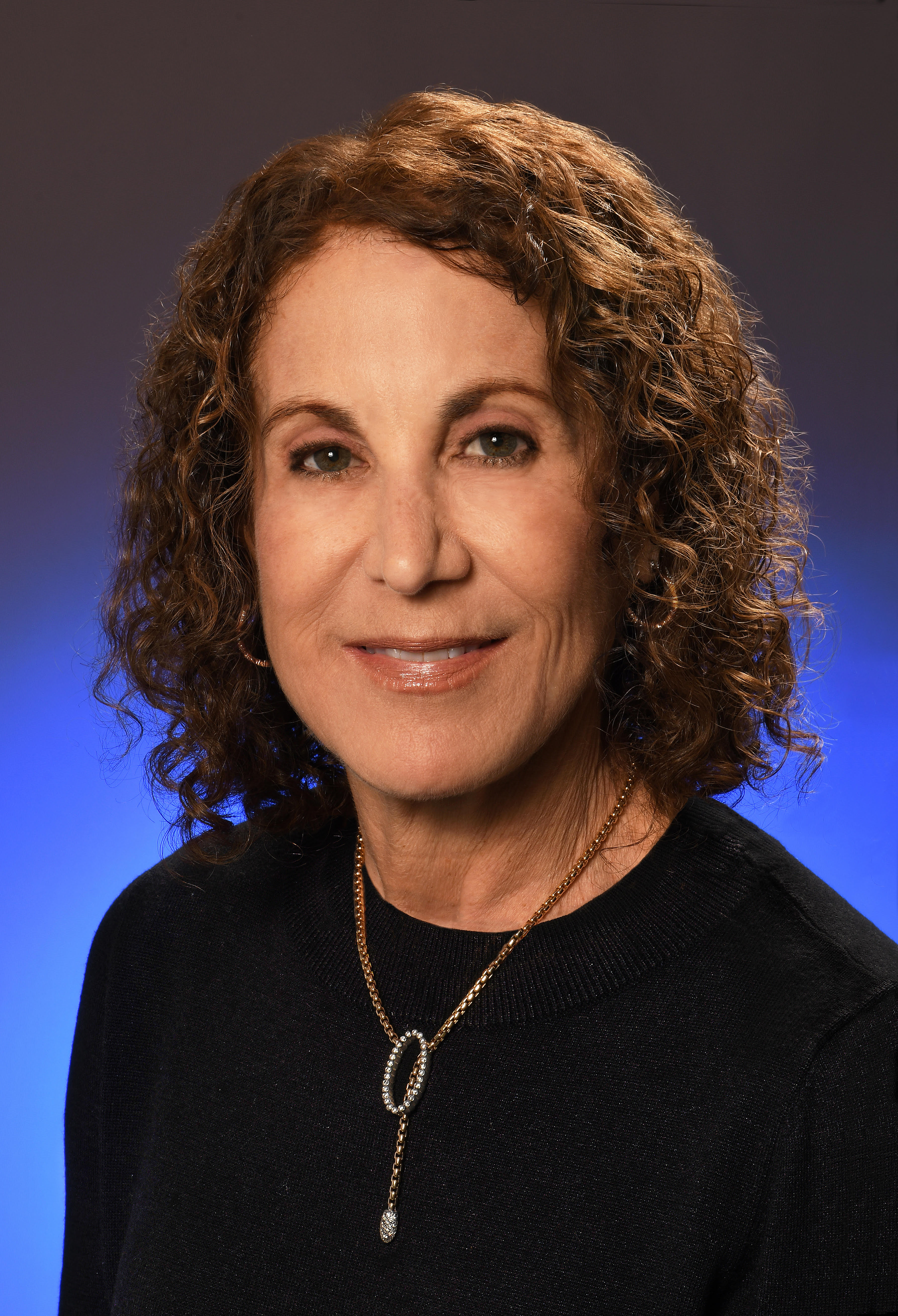Nancy Boguslaw, is Deputy Director of the Maryland/Israel Development Center (MIDC)

Panelists: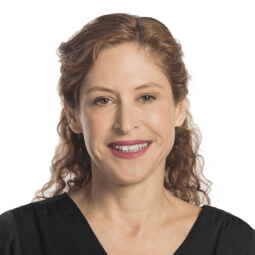Vered Kaplan, CEO of Orgenesis Inc.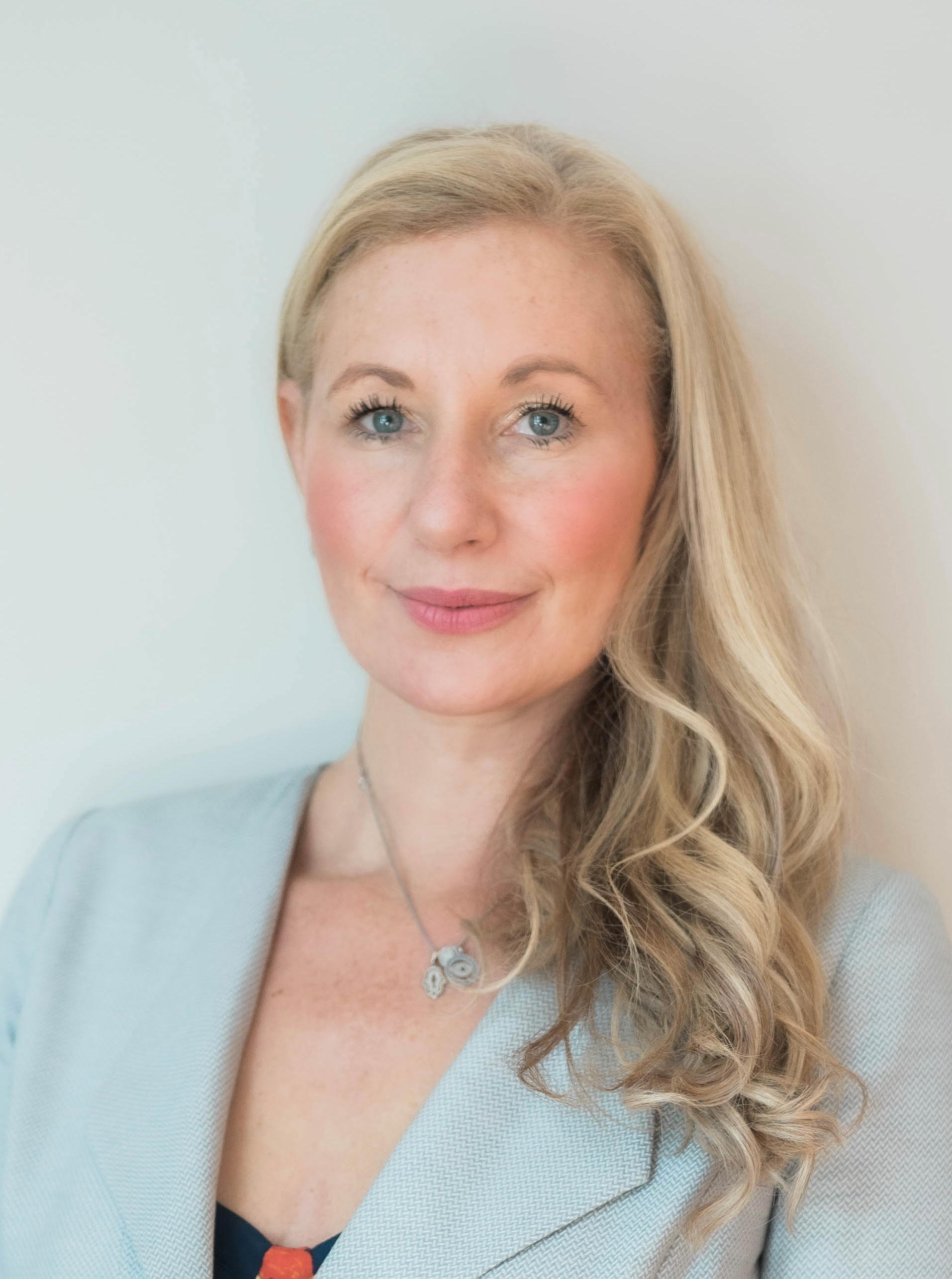Carly Furman, CEO of Nayax LLC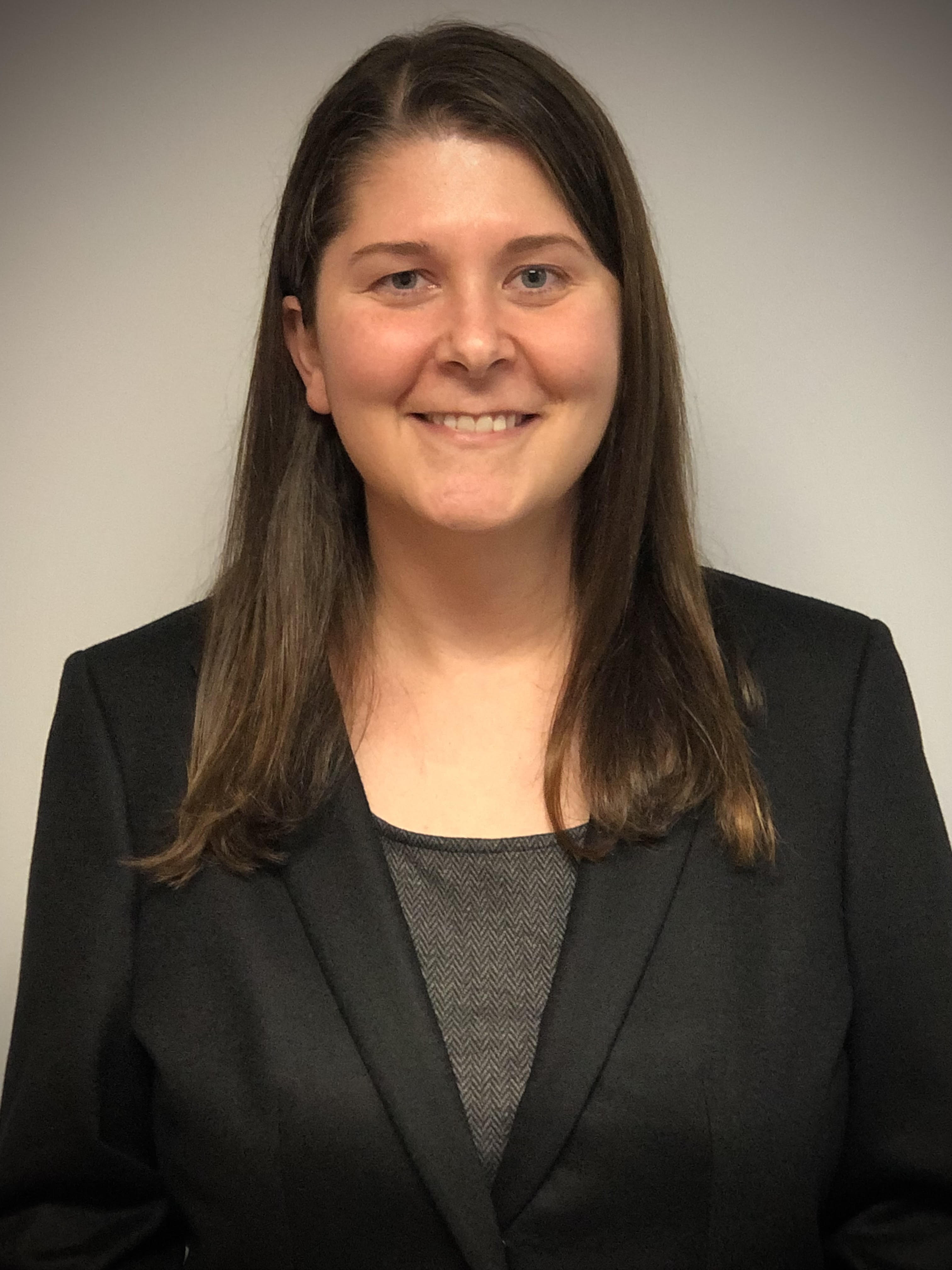Jennifer Roby, is Vice President and CFO at ELTA North America (ENA)

<<<<<<<<<<<<<<<<<<<<<<<<<<<<<<<<<<<<<<<<<<<<<<<<<<<<<
What is Serv-A?

<<<<<<<<<<<<<<<<<<<<<<<<<<<<<<<<<<<<<<<<<<<<<<<<<<<<<

President, CeCe Rund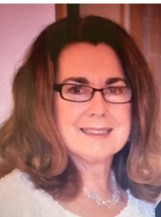<<<<<<<<<<<<<<<<<<<<<<<<<<<<<<<<<<<<<<<<<<<<<<<<<<<<<

Officers for 2023
President - CeCe Rund
Vice President - Julie Bernstein
Vice President - Shulamit Finkelstein
Vice President - Edie Meyers
Corresponding Secretaries - Rochelle Bohrer, Ferne Rogow
Treasurer - Ann Fishkin
Auditor - Rosalind Asch
Community Representative - Marcia Bornfirend, Past President

<<<<<<<<<<<<<<<<<<<<<<<<<<<<<<<<<<<<<<<<<<<<<<<<<<<<<

 The Federation of Jewish Women's Organizations of Maryland is the Oldest Jewish Women's Federation in the Country Founded 1916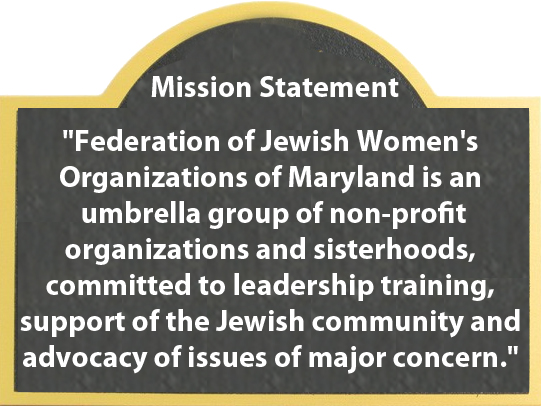Calendar To see the full calendar and Jewish holidays, CLICK HERE.